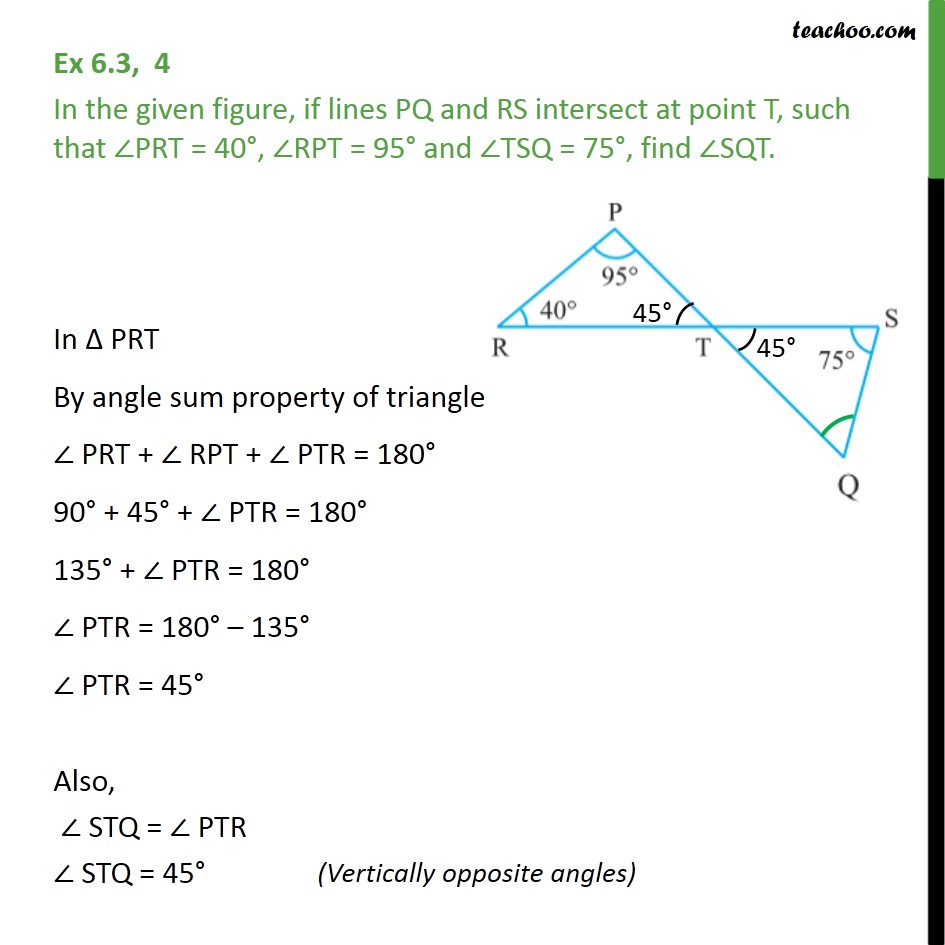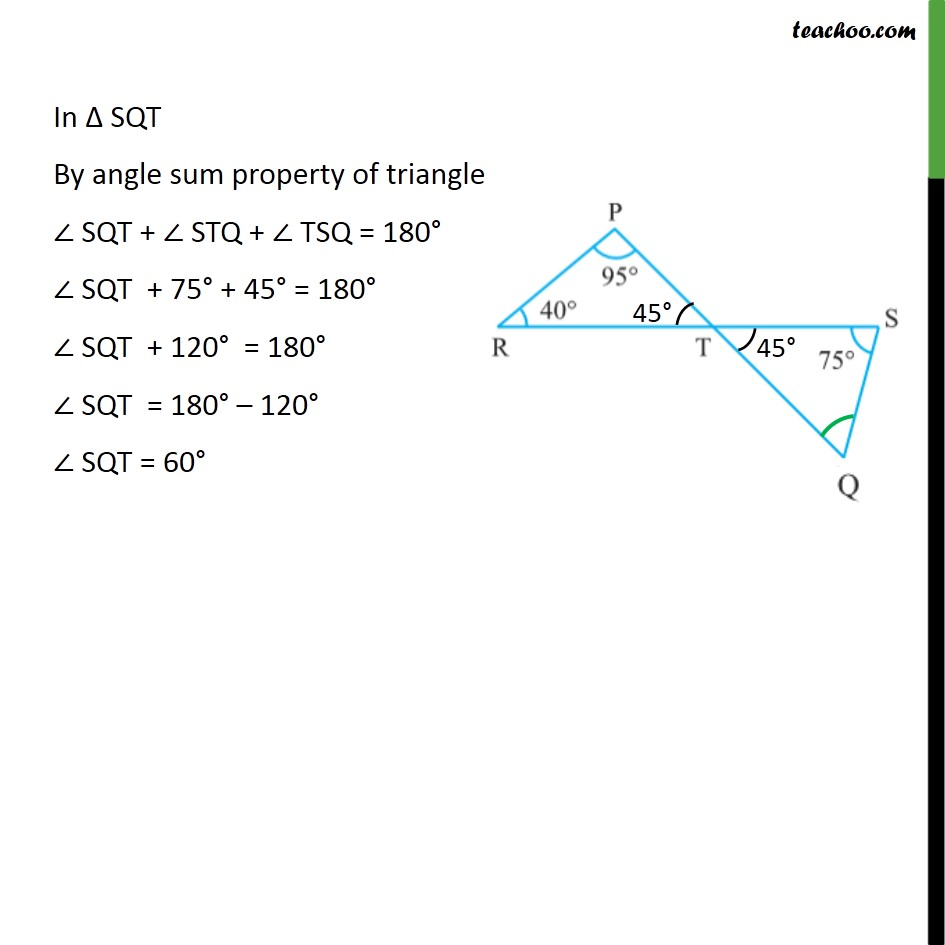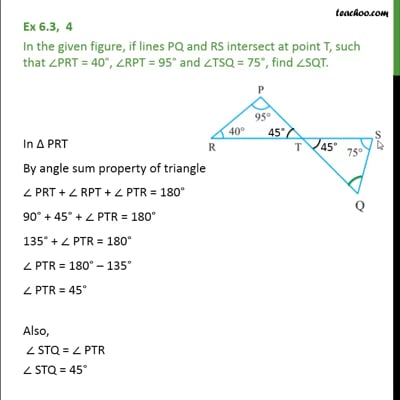Ex 6.3

Chapter 6 Class 9 Lines and Angles
Serial order wise

###googletag.cmd.push(function() { googletag.display('div-gpt-ad-1669298377854-0'); });(adsbygoogle = window.adsbygoogle || []).push({});This video is only available for Teachoo black users

Maths Crash Course - Live lectures + all videos + Real time Doubt solving!

### Transcript

Ex 6.3, 4 In the given figure, if lines PQ and RS intersect at point T, such that PRT = 40 , RPT = 95 and TSQ = 75 , find SQT. In PRT By angle sum property of triangle PRT + RPT + PTR = 180 90 + 45 + PTR = 180 135 + PTR = 180 PTR = 180 135 PTR = 45 Also, STQ = PTR STQ = 45 In SQT By angle sum property of triangle SQT + STQ + TSQ = 180 SQT + 75 + 45 = 180 SQT + 120 = 180 SQT = 180 120 SQT = 60